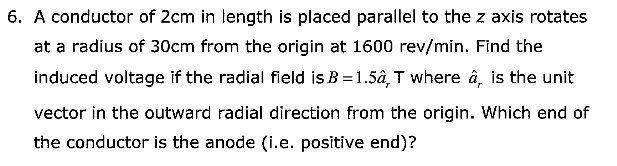# Current induced in rotating conductor in Magnetic Field

• Jimmeh

## Homework Statement## Homework Equations

$l = 0.02 m$

$r = 0.3 m$

$E = \frac{-d\phi}{dt}$

$\phi = BA$

## The Attempt at a Solution

$A = \pi 0.32^2 - \pi 0.3^2 = 0.0124\pi$ ...Area "cut" by conductor

$\phi = 1.5a_r0.0124\pi = 0.0186\pi a_r$ ...change in flux in one revolution

$1600 rev/min = 1600 rev/ 60 seconds = 1 rev / \frac{60}{1600} seconds$

$E = \frac{-0.0186\pi a_r}{\frac{60}{1600}}$

$E = -0.496\pi a_r V$

I'd just like someone to verify this please, and also I'm not sure about which end would be the anode. I think it'd be the end closest the origin, since the magnetic field is in the outward radial direction, $a_r$, the emf induced is in the $-a_r$ direction, and thus current would be flowing towards the origin, but I'm not sure if I went about handling the direction vectors the right way.